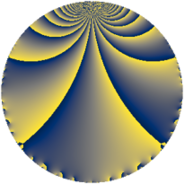# Properties

 Label 5520.2.a.bqLevel $5520$ Weight $2$ Character orbit 5520.a Self dual yes Analytic conductor $44.077$ Analytic rank $1$ Dimension $2$ CM no Inner twists $1$

# Learn more about

## Newspace parameters

 Level: $$N$$ $$=$$ $$5520 = 2^{4} \cdot 3 \cdot 5 \cdot 23$$ Weight: $$k$$ $$=$$ $$2$$ Character orbit: $$[\chi]$$ $$=$$ 5520.a (trivial)

## Newform invariants

 Self dual: yes Analytic conductor: $$44.0774219157$$ Analytic rank: $$1$$ Dimension: $$2$$ Coefficient field: $$\Q(\sqrt{33})$$ Defining polynomial: $$x^{2} - x - 8$$ Coefficient ring: $$\Z[a_1, \ldots, a_{7}]$$ Coefficient ring index: $$1$$ Twist minimal: no (minimal twist has level 2760) Fricke sign: $$1$$ Sato-Tate group: $\mathrm{SU}(2)$

## $q$-expansion

Coefficients of the $$q$$-expansion are expressed in terms of $$\beta = \frac{1}{2}(1 + \sqrt{33})$$. We also show the integral $$q$$-expansion of the trace form.

 $$f(q)$$ $$=$$ $$q + q^{3} + q^{5} -\beta q^{7} + q^{9} +O(q^{10})$$ $$q + q^{3} + q^{5} -\beta q^{7} + q^{9} -4 q^{11} + ( -2 + 2 \beta ) q^{13} + q^{15} + ( 2 + \beta ) q^{17} -4 q^{19} -\beta q^{21} - q^{23} + q^{25} + q^{27} + ( -2 - \beta ) q^{29} -\beta q^{31} -4 q^{33} -\beta q^{35} + ( -2 - \beta ) q^{37} + ( -2 + 2 \beta ) q^{39} + ( 2 - 3 \beta ) q^{41} + 4 q^{43} + q^{45} + 2 \beta q^{47} + ( 1 + \beta ) q^{49} + ( 2 + \beta ) q^{51} + ( -2 + \beta ) q^{53} -4 q^{55} -4 q^{57} + ( -12 + \beta ) q^{59} -2 q^{61} -\beta q^{63} + ( -2 + 2 \beta ) q^{65} + ( -12 - \beta ) q^{67} - q^{69} -\beta q^{71} + ( -6 - 2 \beta ) q^{73} + q^{75} + 4 \beta q^{77} + 4 \beta q^{79} + q^{81} + ( -4 + 5 \beta ) q^{83} + ( 2 + \beta ) q^{85} + ( -2 - \beta ) q^{87} + ( 2 - 2 \beta ) q^{89} -16 q^{91} -\beta q^{93} -4 q^{95} + ( 2 + 4 \beta ) q^{97} -4 q^{99} +O(q^{100})$$ $$\operatorname{Tr}(f)(q)$$ $$=$$ $$2q + 2q^{3} + 2q^{5} - q^{7} + 2q^{9} + O(q^{10})$$ $$2q + 2q^{3} + 2q^{5} - q^{7} + 2q^{9} - 8q^{11} - 2q^{13} + 2q^{15} + 5q^{17} - 8q^{19} - q^{21} - 2q^{23} + 2q^{25} + 2q^{27} - 5q^{29} - q^{31} - 8q^{33} - q^{35} - 5q^{37} - 2q^{39} + q^{41} + 8q^{43} + 2q^{45} + 2q^{47} + 3q^{49} + 5q^{51} - 3q^{53} - 8q^{55} - 8q^{57} - 23q^{59} - 4q^{61} - q^{63} - 2q^{65} - 25q^{67} - 2q^{69} - q^{71} - 14q^{73} + 2q^{75} + 4q^{77} + 4q^{79} + 2q^{81} - 3q^{83} + 5q^{85} - 5q^{87} + 2q^{89} - 32q^{91} - q^{93} - 8q^{95} + 8q^{97} - 8q^{99} + O(q^{100})$$

## Embeddings

For each embedding $$\iota_m$$ of the coefficient field, the values $$\iota_m(a_n)$$ are shown below.

For more information on an embedded modular form you can click on its label.

Label $$\iota_m(\nu)$$ $$a_{2}$$ $$a_{3}$$ $$a_{4}$$ $$a_{5}$$ $$a_{6}$$ $$a_{7}$$ $$a_{8}$$ $$a_{9}$$ $$a_{10}$$
1.1
 3.37228 −2.37228
0 1.00000 0 1.00000 0 −3.37228 0 1.00000 0
1.2 0 1.00000 0 1.00000 0 2.37228 0 1.00000 0
 $$n$$: e.g. 2-40 or 990-1000 Significant digits: Format: Complex embeddings Normalized embeddings Satake parameters Satake angles

## Atkin-Lehner signs

$$p$$ Sign
$$2$$ $$1$$
$$3$$ $$-1$$
$$5$$ $$-1$$
$$23$$ $$1$$

## Inner twists

This newform does not admit any (nontrivial) inner twists.

## Twists

By twisting character orbit
Char Parity Ord Mult Type Twist Min Dim
1.a even 1 1 trivial 5520.2.a.bq 2
4.b odd 2 1 2760.2.a.o 2
12.b even 2 1 8280.2.a.z 2

By twisted newform orbit
Twist Min Dim Char Parity Ord Mult Type
2760.2.a.o 2 4.b odd 2 1
5520.2.a.bq 2 1.a even 1 1 trivial
8280.2.a.z 2 12.b even 2 1

## Hecke kernels

This newform subspace can be constructed as the intersection of the kernels of the following linear operators acting on $$S_{2}^{\mathrm{new}}(\Gamma_0(5520))$$:

 $$T_{7}^{2} + T_{7} - 8$$ $$T_{11} + 4$$ $$T_{13}^{2} + 2 T_{13} - 32$$ $$T_{17}^{2} - 5 T_{17} - 2$$ $$T_{19} + 4$$

## Hecke characteristic polynomials

$p$ $F_p(T)$
$2$ $$T^{2}$$
$3$ $$( -1 + T )^{2}$$
$5$ $$( -1 + T )^{2}$$
$7$ $$-8 + T + T^{2}$$
$11$ $$( 4 + T )^{2}$$
$13$ $$-32 + 2 T + T^{2}$$
$17$ $$-2 - 5 T + T^{2}$$
$19$ $$( 4 + T )^{2}$$
$23$ $$( 1 + T )^{2}$$
$29$ $$-2 + 5 T + T^{2}$$
$31$ $$-8 + T + T^{2}$$
$37$ $$-2 + 5 T + T^{2}$$
$41$ $$-74 - T + T^{2}$$
$43$ $$( -4 + T )^{2}$$
$47$ $$-32 - 2 T + T^{2}$$
$53$ $$-6 + 3 T + T^{2}$$
$59$ $$124 + 23 T + T^{2}$$
$61$ $$( 2 + T )^{2}$$
$67$ $$148 + 25 T + T^{2}$$
$71$ $$-8 + T + T^{2}$$
$73$ $$16 + 14 T + T^{2}$$
$79$ $$-128 - 4 T + T^{2}$$
$83$ $$-204 + 3 T + T^{2}$$
$89$ $$-32 - 2 T + T^{2}$$
$97$ $$-116 - 8 T + T^{2}$$
show more
show less